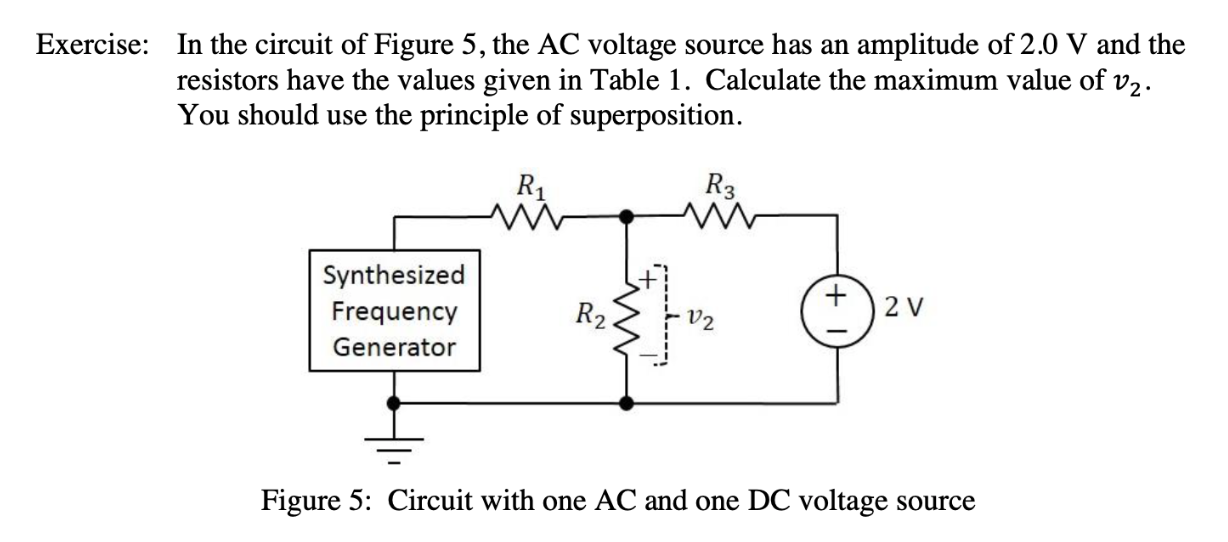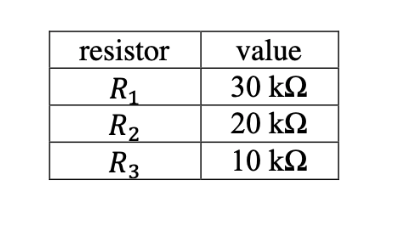# In the circuit of Figure 5, the AC voltage source has anresistors have the values given in Table 1. Calculate the maximum value of v2You should use the principle of superpositionExercise:amplitude of 2.0 V and theR3Synthesized2 VFrequencyR2GeneratorFigure 5: Circuit with one AC and one DC voltageSource+ resistorvalue30 k220 kΩR2R310 k2

Question
75 viewshelp_outlineImage TranscriptioncloseIn the circuit of Figure 5, the AC voltage source has an resistors have the values given in Table 1. Calculate the maximum value of v2 You should use the principle of superposition Exercise: amplitude of 2.0 V and the R3 Synthesized 2 V Frequency R2 Generator Figure 5: Circuit with one AC and one DC voltage Source + fullscreenhelp_outlineImage Transcriptioncloseresistor value 30 k2 20 kΩ R2 R3 10 k2 fullscreen
check_circle

Step 1

Synthesized frequency generator- A frequency synthesizer is an electronic circuit that generates a range of frequencies from a single reference frequency. In a network it can be used as an AC source with a desired frequency.

Step 2

The following figure has one AC source and one DC source. Frequency synthesizer is considered as an AC source with 2 V amplitude

Step 3

Superposition theorem can be used to find out the maximum voltage across R2. Superposition theorem states that in a linear, active, bilateral network if two or more than two independent sources are present the...

### Want to see the full answer?

See Solution

#### Want to see this answer and more?

Solutions are written by subject experts who are available 24/7. Questions are typically answered within 1 hour.*

See Solution
*Response times may vary by subject and question.
Tagged in

### Electrical Engineering